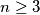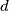### IMO Shortlist 1965 problem 6

Kvaliteta:
Avg: 0.0
Težina:
Avg: 0.0
In a plane a set of$n$ points ($n \geq 3$) is give. Each pair of points is connected by a segment. Let$d$ be the length of the longest of these segments. We define a diameter of the set to be any connecting segment of length$d$. Prove that the number of diameters of the given set is at most$n$.
Source: Međunarodna matematička olimpijada, shortlist 1965